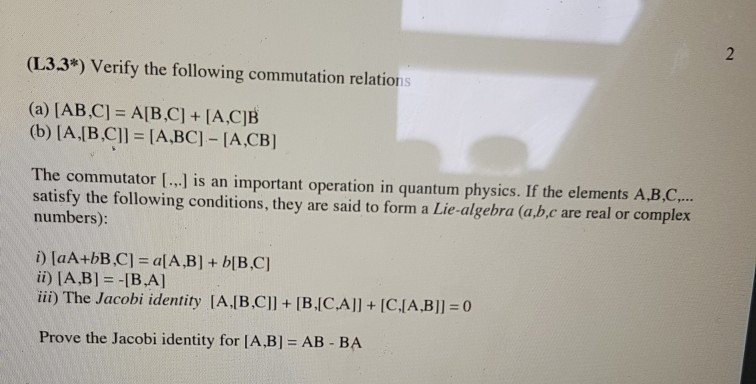# (L33*) Verify the following commutation relations (a) [AB,C] A[B,C +IA,CIB (b) [A,|B C]l-IA,BC] [A,CB] The commutato...

###### Question:(L33*) Verify the following commutation relations (a) [AB,C] A[B,C +IA,CIB (b) [A,|B C]l-IA,BC] [A,CB] The commutator [..] is an important operation in quantum physics. If the elements A.B.C... satisfy the following conditions, they are said to form a Lie-algebra (ab,c are real or complex numbers): ii) [A,Bl -IB.Al iii) The Jacobi identity [AJB CII + [BJCA]] + [CJA,BII :0 - BA Prove the Jacobi identity for [A,B] AB

#### Similar Solved Questions

##### What are the missing reagents, products, appropriate coefficients, and states for the reaction of potassium with...
What are the missing reagents, products, appropriate coefficients, and states for the reaction of potassium with water. [...] denotes missing component [...] K[...] + 2 H2O[...] --> [...] K+[...] + 2 OH (aq) + [...] (g) 2; (s); (I); 2; (aq); H2 2; (aq); (aq); 2; (aq); H2 1; (s); (aq); 1; (aq); O2...
##### For the one-dimensional particle in a box of length L = 1 Å, what will be...
For the one-dimensional particle in a box of length L = 1 Å, what will be the energy of the ground state? a. Write Schrodinger’s equation for if the potential between 0 and L is zero b. Write Schrodinger’s equation for if the potential between 0 and L has a constant value of V_o...
##### If the active power consumed by the load is 8 kW and the reactive power consumed...
If the active power consumed by the load is 8 kW and the reactive power consumed by the load is 6 kVAR, the load power factor is: Select one: 0.75 1.25 0.8 0.6...